# 45 Datasets

 45 Datasets
Especially in larger organizations, computing often centers around dealing with large amounts of structured data. The Wolfram Language has a very powerful way to deal with structured data, using what it calls datasets.
A simple example of a dataset is formed from an association of associations.
Create a simple dataset that can be viewed as having 2 rows and 3 columns:
data = Dataset[<|"a" -> <|"x" -> 1, "y" -> 2, "z" -> 3|>, "b" -> <|"x" -> 5, "y" -> 10, "z" -> 7|>|>]

The Wolfram Language displays most datasets in tabular form. You can extract parts from datasets just like you would from associations.
Get the element from “row b” and “column z”:
data["b", "z"]

You can first extract the whole “b row”, then get the “z” element of the result:
data["b"]["z"]

You can also just get the whole “b row” of the dataset. The result is a new dataset, which for ease of reading happens to be displayed in this case as a column.
Generate a new dataset from the “b row” of the original dataset:
data["b"]

Here is the dataset that corresponds to the “z column” for all “rows”.
Generate a dataset consisting of the “z column” for all rows:
data[All, "z"]

Get totals for each row by applying Total to all columns for all the rows:
data[All, Total]

If we use f instead of Total, we can see what’s going on: the function is being applied to each of the “row” associations.
Apply the function f to each row:
data[All, f]

Apply a function that adds the x and z elements of each association:
data[All, #x + #z &]

You can use any function; here’s PieChart:
data[All, PieChart]

You can give a function to apply to all rows too.
This extracts the value of each “z column”, then applies f to the association of results:
data[f, "z"]

Apply f to the totals of all columns:
data[f, Total]

data[Max, Total]

You can always “chain” queries, for example first finding the totals for all rows, then picking out the result for the “b row”.
Find totals for all rows, then pick out the total for the “b row”:
data[All, Total]["b"]

It’s equivalent to this:
data["b", Total]

Select numbers greater than 5 from a list:
Select[{1, 3, 6, 8, 2, 5, 9, 7}, # > 5 &]

Another way to get the same answer, using the operator form of Select:
Select[# > 5 &][{1, 3, 6, 8, 2, 5, 9, 7}]

The operator form of Select is a function which can be applied to actually perform the Select operation.
Make a dataset by selecting only rows whose “z column” is greater than 5:
data[Select[#z > 5 &]]

For each row, select columns whose values are greater than 5, leaving a ragged structure:
data[All, Select[# > 5 &]]

Normal turns the dataset into an ordinary association of associations:
Normal[%]

Many Wolfram Language functions have operator forms.
Sort according to the values of a function applied to each element:
SortBy[{1, 3, 6, 8, 2, 5, 9, 7}, If[EvenQ[#], #, 10 + #] &]

SortBy has an operator form:
SortBy[If[EvenQ[#], #, 10 + #] &][{1, 3, 6, 8, 2, 5, 9, 7}]

Sort rows according to the value of the difference of the x and y columns:
data[SortBy[#x - #y &]]

Sort the rows, and find the total of all columns:
data[SortBy[#x - #y &], Total]

Sometimes you want to apply a function to each element in the dataset.
Apply f to each element in the dataset:
data[All, All, f]

Sort the rows before totaling the squares of their elements:
data[SortBy[#x - #y &], Total, #^2 &]

A dataset formed from a list of associations:
Dataset[{<|"x" -> 2, "y" -> 4, "z" -> 6|>, <|"x" -> 11, "y" -> 7, "z" -> 1|>}]

It’s OK for entries to be missing:
Dataset[{<|"x" -> 2, "y" -> 4, "z" -> 6|>, <|"x" -> 11, "y" -> 7|>}]

Now that we’ve seen some simple examples, it’s time to look at something slightly more realistic. Let’s import a dataset giving properties of planets and moons. The dataset has a hierarchical structure, with each planet having a mass and radius of its own, and then also having a collection of moons, each of which have their own properties. This general structure is extremely common in practice (think students and grades, customers and orders, etc.).
Get a hierarchical dataset of planets and moons from the cloud:
planets = CloudGet["http://wolfr.am/7FxLgPm5"]

Make a bar chart of planet radii:

If we ask about the moons of Mars, we get a dataset, which we can then query further.
Get a dataset about the moons of Mars:
planets["Mars", "Moons"]

“Drill down” to make a table of radii of all the moons of Mars:

planets[All, "Moons", Length]

Find the total mass of all moons for each planet:
planets[All, "Moons", Total, "Mass"]

Get the same result, but only for planets with more than 10 moons:
planets[Select[Length[#Moons] > 10 &], "Moons", Total, "Mass"]

Make a pie chart of the result:
PieChart[%, ChartLegends -> Automatic]

For all moons, select ones whose mass is greater than 0.01 times the mass of the Earth:
planets[All, "Moons", Select[#Mass > Quantity[0.01, "EarthMass"] &]]

Get the list of keys (i.e. moon names) in the resulting association for each planet:
planets[All, "Moons", Select[#Mass > Quantity[0.01, "EarthMass"] &]][All, Keys]

Get the underlying association:
Normal[%]

Join together, or “catenate”, the lists for all keys:
Catenate[%]

Here’s the whole computation in one line:
planets[All, "Moons", Select[#Mass > Quantity[0.01, "EarthMass"] &]][ Catenate, Keys] // Normal

Make number line plots of the logarithms of masses for moons of each planet:
planets[All, "Moons", NumberLinePlot[Values[#]] &, Log[#Mass/Quantity[1, "EarthMass"]] &]

As a final example, let’s make a word cloud of names of moons, sized according to the masses of the moons. To do this, we need a single association that associates the name of each moon with its mass.
When given an association, WordCloud determines sizes from values in the association:
WordCloud[<|"A" -> 5, "B" -> 4, "C" -> 3, "D" -> 2, "E" -> 1|>]

The function Association combines associations:
Association[<|"a" -> 1, "b" -> 2|>, <|"c" -> 3|>]

Generate the word cloud of moon masses:
planets[WordCloud[Association[Values[#]]] &, "Moons", All, "Mass"]

We’ve seen before that we can write something like f[g[x]] as f@g@x or x//g//f. We can also write it f[g[#]]&[x]. But what about f[g[#]]&? Is there a short way to write this? The answer is that there is, in terms of the function composition operators @* and /*.
f@*g@*h represents a composition of functions to be applied right-to-left:
(f@*g@*h)[x]

h/*g/*f represents a composition of functions to be applied left-to-right:
(h/*g/*f)[x]

Here’s the previous code rewritten using composition @*:
planets[WordCloud@*Association@*Values, "Moons", All, "Mass"]

And using right composition /*:
planets[Values/*Association/*WordCloud, "Moons", All, "Mass"]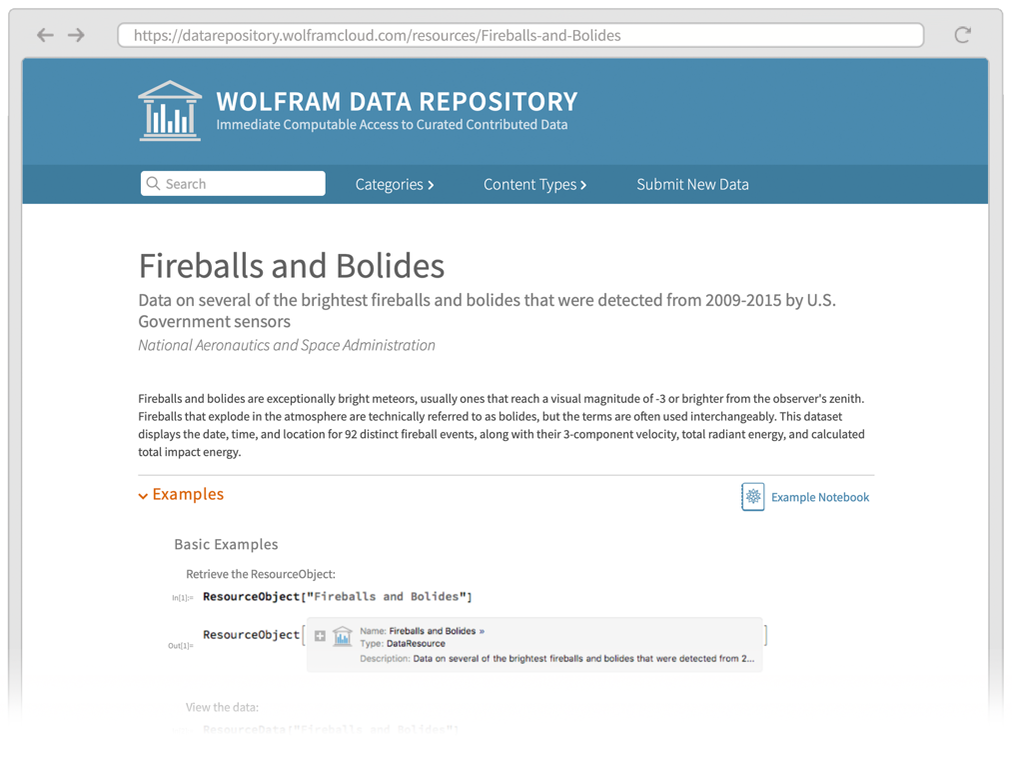To get the main dataset that’s mentioned here, just use ResourceData.
Get the dataset just by giving its name to ResourceData:
fireballs = ResourceData["Fireballs and Bolides"]

GeoListPlot[fireballs[All, "Coordinates"]]

Make a histogram of the altitudes:
Histogram[fireballs[All, "Altitude"]]

 Dataset[data] a dataset Normal[dataset] convert a dataset to normal lists and associations Catenate[{assoc1, ...}] catenate associations, combining their elements f@*g composition of functions ( f[g[x]] when applied to x) f/*g right composition (g[f[x]] when applied to x)
Note: These exercises use the dataset planets=CloudGet["https://wolfr.am/7FxLgPm5"].
45.1Make a word cloud of the planets, with weights determined by their number of moons. »
Sample expected output:
 Out[]=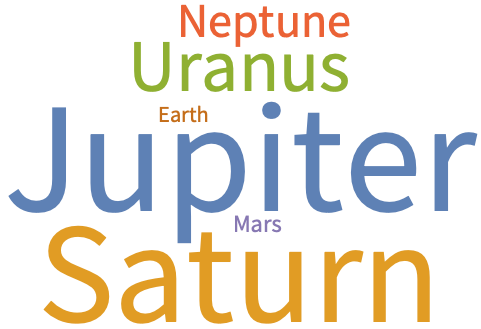Answer & check your solution
45.2Make a bar chart of the number of moons for each planet. »
Sample expected output:
 Out[]=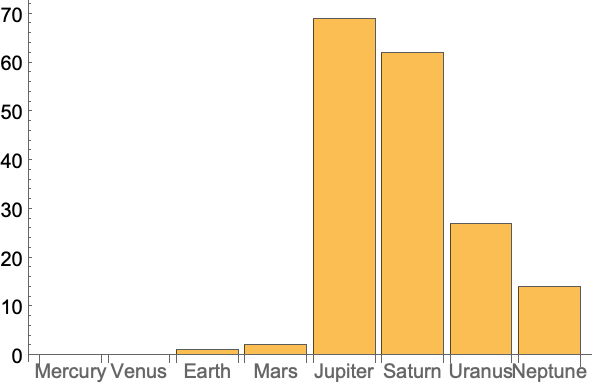Answer & check your solution
45.3Make a dataset of the masses of the planets, sorted by their number of moons. »
Sample expected output:
 Out[]=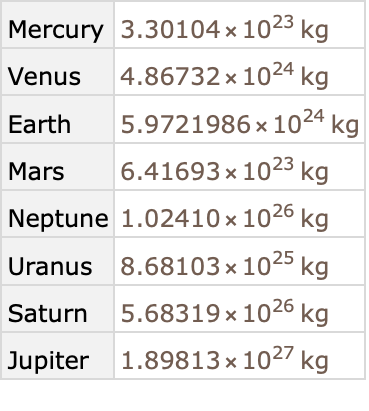Answer & check your solution
45.4Make a dataset of planets and the mass of each one’s most massive moon. »
Sample expected output:
 Out[]=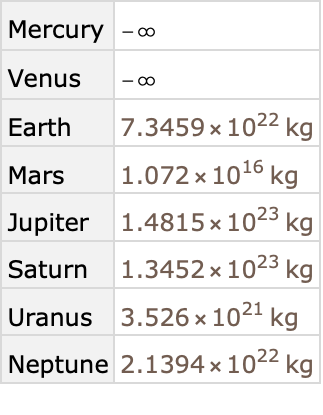Answer & check your solution
Sample expected output:
 Out[]=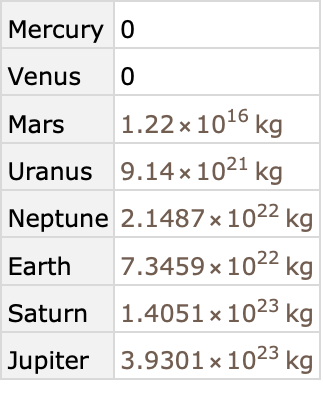Answer & check your solution
Sample expected output:
 Out[]=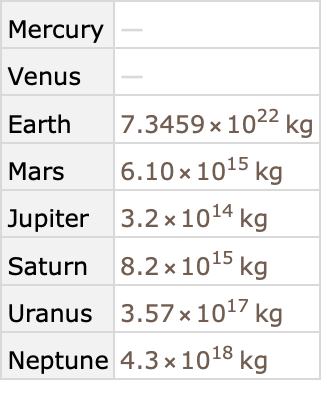Answer & check your solution
45.7For each planet, make a list of moons larger in mass than 0.0001 Earth masses. »
Sample expected output:
 Out[]=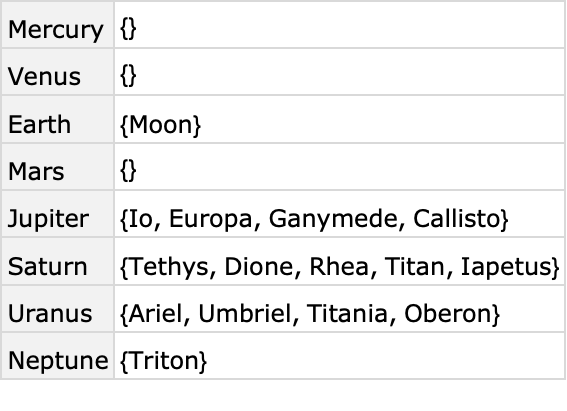Answer & check your solution
45.8Make a word cloud of countries in Central America, with the names of countries proportional to the lengths of the Wikipedia article about them. »
Sample expected output:
 Out[]=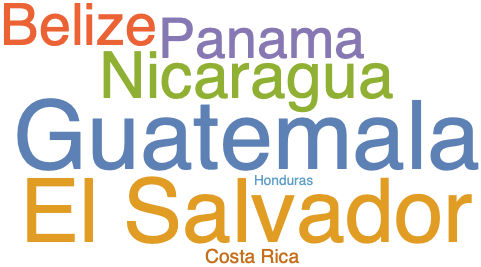Answer & check your solution
45.9Find the maximum observed altitude in the Fireballs & Bolides dataset. »
Expected output:
 Out[]=Answer & check your solution
45.10Find a dataset of the 5 largest observed altitudes in the Fireballs & Bolides dataset. »
Expected output:
 Out[]=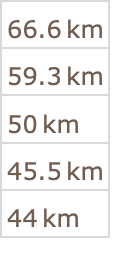Answer & check your solution
45.11Make a histogram of the differences in successive peak brightness times in the Fireballs & Bolides dataset. »
Expected output:
 Out[]=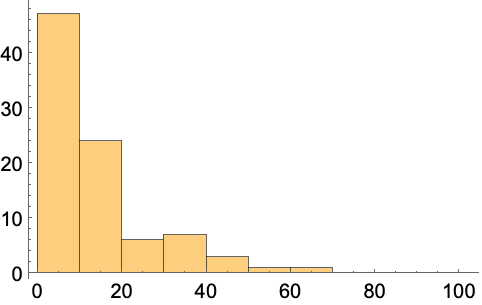Answer & check your solution
45.12Plot the nearest cities for the first 10 entries in the Fireballs & Bolides dataset, labeling each city. »
Expected output:
 Out[]=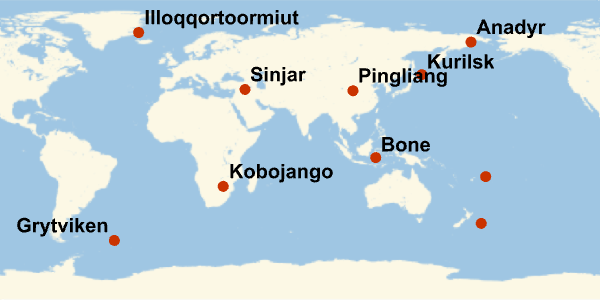Answer & check your solution
45.13Plot the nearest cities for the 10 entries with largest altitudes in the Fireballs & Bolides dataset, labeling each city. »
Expected output:
 Out[]=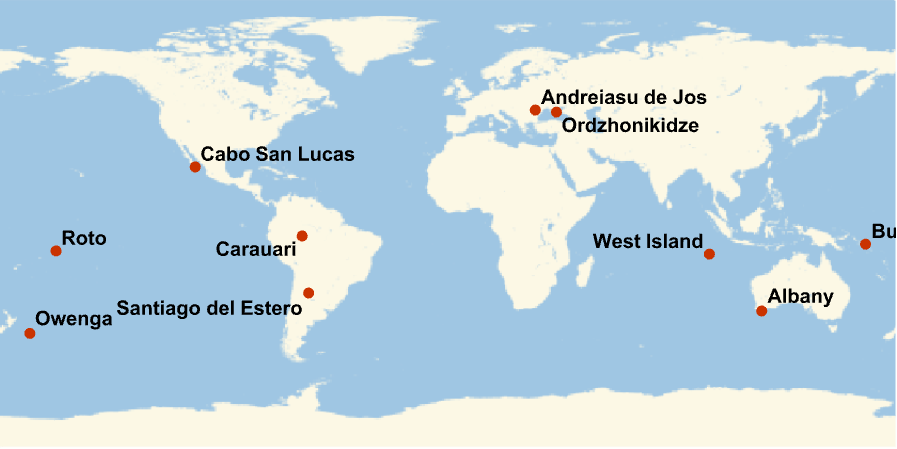Answer & check your solution
What kinds of data can datasets contain?
Any kinds. Not just numbers and text but also images, graphs and lots more. There’s no need for all elements of a particular row or column to be the same type.
Can I adjust the formatting of datasets?
Yes. There are many options. For example, Dataset[dataset, MaxItems 5] will display 5 items per “page”.
Can I turn spreadsheets into datasets?
Yes. SemanticImport is often a good way to do it.
What are databases and how do they relate to Dataset?
Databases are a traditional way to store structured data in a computer system. Databases are often set up to allow both reading and writing of data. Dataset is a way to represent data that might be stored in a database so that it’s easy to manipulate with the Wolfram Language.
How does data in Dataset compare to data in an SQL (relational) database?
SQL databases are strictly based on tables of data arranged in rows and columns of particular types, with additional data linked in through “foreign keys”. Dataset can have any mixture of types of data, with any number of levels of nesting, and any hierarchical structure, somewhat more analogous to a NoSQL database, but with additional operations made possible by the symbolic nature of the language.
Can I use datasets to set up entities and values for them?
• Dataset supports a new kind of symbolic database structure which generalizes both relational and hierarchical databases.
• Dataset has many additional mechanisms and capabilities that we haven’t discussed.
• Everything that can be done with queries on datasets can also be done by using functions like Map and Apply on underlying lists and associationbut it’s typically much simpler with dataset queries.
• You can connect the Wolfram Language directly to SQL databasesand do queries with SQL syntaxusing DatabaseLink.